# <transform-function>

<transform-function> CSS数据类型用于对元素的显示做变换。通常，这种变换可以由矩阵表示，并且可以使用每个点上的矩阵乘法来确定所得到的图像。

## 浏览器支持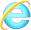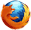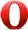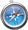浏览器都支持``数据类型

## 2D图形的坐标系统

### 笛卡尔坐标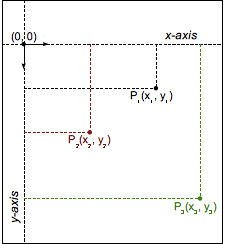```a   c
b   d
```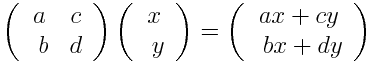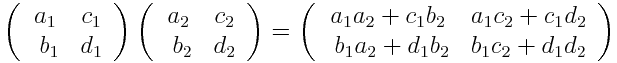## matrix()

CSS 函数 matrix() 用六个指定的值来指定一个均匀的二维（2D）变换矩阵。这个矩形中的常量值是不作为参数进行传递的，其他的参数则在主要列的顺序中描述。

matrix(a, b, c, d, tx, ty) 是 matrix3d(a, b, 0, 0, c, d, 0, 0, 0, 0, 1, 0, tx, ty, 0, 1) 的简写

### 语法

matrix(a, b, c, d, tx, ty)
• a b c d：以 `<number>` 的格式来描述线性变换
• tx ty：以 `<number>` 的格式来描述变换的量

$\left(\begin{array}{cc}a& c\end{array}\right)$
b d
$\left(\begin{array}{ccc}a& c& ty\end{array}\right)$
b d tx
0 0 1
$\left(\begin{array}{ccc}a& c& ty\end{array}\right)$
b d tx
0 0 1
$\left(\begin{array}{cccc}a& c& 0& tx\end{array}\right)$
b d 0 ty
0 0 1 0
0 0 0 1
`[a b c d tx ty]`

## matrix3d()

CSS 函数 matrix3d() 用一个 4 × 4 的齐次矩阵来描述一个三维（3D）变换。16个参数都在主要列的顺序中描述。

### 语法

matrix3d(a1, b1, c1, d1, a2, b2, c2, d2, a3, b3, c3, d3, a4, b4, c4, d4)
• a1, b1, c1, d1, a2, b2, c2, d2, a3, b3, c3, d3, a4, b4, c4, d4：以 `<number>` 的格式来描述线性变换
• a4 b4 c4：以 `<number>` 的格式来描述变换的量

c1 c2 c3 c4
d1 d2 d3 d4

## perspective()

perspective() 这个CSS函数定义了 z=0平面与用户之间的距离，以便给三维定位元素一定透视度。当每个3D元素的z>0时会显得比较大，而在z<0时会显得比较小。其影响的程度由这个属性的值来决定。

### 语法

perspective(<length>)
• <length>：给定从用户（显示屏）到z = 0平面的距离。 它用于将透视图转换应用于元素。 如果它是0或负值，则不应用透视变换。

## rotate()

The rotate() CSS 函数 定义一个旋转属性，将元素在不变形的情况下旋转到不动点周围(如 transform-origin 属性所指定) 。 移动量由指定角度定义;如果为正值，则运动将为顺时针，如果为负值，则为逆时针 。 180°的旋转称为点反射 (point reflection)。### 语法

rotate(<angle>)
• <angle>：代表旋转的角度。正角表示顺时针旋转，负角表示逆时针旋转。
Cartesian coordinates on ℝ2 Homogeneous coordinates on ℝℙ2 Cartesian coordinates on ℝ3 Homogeneous coordinates on ℝℙ3
$\left(\begin{array}{cc}cos\left(a\right)& -sin\left(a\right)\\ sin\left(a\right)& cos\left(a\right)\end{array}\right)$ $\left(\begin{array}{ccc}cos\left(a\right)& -sin\left(a\right)& 0\\ sin\left(a\right)& cos\left(a\right)& 0\\ 0& 0& 1\end{array}\right)$ $\left(\begin{array}{ccc}cos\left(a\right)& -sin\left(a\right)& 0\\ sin\left(a\right)& cos\left(a\right)& 0\\ 0& 0& 1\end{array}\right)$ $\left(\begin{array}{cccc}cos\left(a\right)& -sin\left(a\right)& 0& 0\\ sin\left(a\right)& cos\left(a\right)& 0& 0\\ 0& 0& 1& 0\\ 0& 0& 0& 1\end{array}\right)$
`[cos(a) `sin(a)` -sin(a) cos(a) 0 0]`

## rotate3d()

rotate3d() 这个CSS 函数定义一个3D旋转功能，该旋转使元素能够绕固定轴移动而不变形。 其中移动量由指定角度定义；如果为正值，则运动将为顺时针，如果为负值，则为逆时针。

### 语法

rotate3d(x, y, z, a)
• x：该参数为 `<number>` 类型值，描述旋转轴向量的x坐标 。
• y：该参数为 `<number>` 类型值，描述旋转轴向量的y坐标。
• z：该参数为 `<number>` 类型值，描述旋转轴向量的z坐标。
• a：该参数为 `<angle>` 代表旋转的角度 。 正角度表示顺时针旋转，负角度表示逆时针旋转 。
Cartesian coordinates on ℝ2 Homogeneous coordinates on ℝℙ2 Cartesian coordinates on ℝ3 Homogeneous coordinates on ℝℙ3

## rotateX()

rotateX() 这个CSS 函数定义了将元素在横坐标上旋转而不使其变形的方法。 其运动的程度由指定的角度来定义；如果是正的，则为顺时针旋转，如果是负的，则是逆时针旋转。

rotateX(a)是 rotate3D(1, 0, 0, a)的简写形式。

### 语法

rotateX(<angle>)
• <angle>：代表旋转的角度。正角表示顺时针旋转，负角表示逆时针旋转。
Cartesian coordinates on ℝ2 Homogeneous coordinates on ℝℙ2 Cartesian coordinates on ℝ3 Homogeneous coordinates on ℝℙ3

## rotateY()

rotateY() 这个CSS 函数定义了将元素在纵坐标上旋转而不使其变形的方法。 其运动的程度由指定的角度来定义；如果是正的，则为顺时针旋转，如果是负的，则是逆时针旋转。

rotateY(a) 是 rotate3D(0, 1, 0, a) 的简写形式。

### 语法

rotateY(<angle>)
• <angle>：代表旋转的角度。正角表示顺时针旋转，负角表示逆时针旋转。
Cartesian coordinates on ℝ2 Homogeneous coordinates on ℝℙ2 Cartesian coordinates on ℝ3 Homogeneous coordinates on ℝℙ3

## rotate

rotateZ()这个CSS 函数定义了将元素在z轴上旋转而不使其变形的方法。 其运动的程度由指定的角度来定义；如果是正的，则为顺时针旋转，如果是负的，则是逆时针旋转。

rotateZ(a) 是 rotate3D(0, 0, 1, a) 的简写形式。

### 语法

rotateZ(<angle>)
• <angle>：代表旋转的角度。正角表示顺时针旋转，负角表示逆时针旋转。
Cartesian coordinates on ℝ2 Homogeneous coordinates on ℝℙ2 Cartesian coordinates on ℝ3 Homogeneous coordinates on ℝℙ3

## scale()

The scale() CSS 函数可改变元素的大小。 它可以增大或减小元素的大小，并且缩放量由矢量定义，并且它可以使在一个方向上比另一个方向更多。这种变换的特点是矢量的坐标可定义在每个不同方向上各子完成一定比例缩放。如果矢量的两个坐标相等，则缩放是均匀的或各向同性的，并且元素的形状被保留。在这种情况下，缩放函数定义了一个同调变换。### 语法

scale(sx | sx, sy)
• sx：该参数为 `<number>` 类型值，代表缩放矢量的横坐标。
• sy：该参数为 `<number>` 类型值，代表缩放矢量的纵坐标 。 如果不存在，则其默认值为 sx，从而导致保持元素形状进行均匀缩放。

## scale3d()

`scale3d()` CSS 函数可改变元素的大小。由于缩放的量由矢量定义，因此可以改变不同方向的尺寸。

### 语法

scale3d(sx, sy, sz)

### 参数

sx

sy

sz

Cartesian coordinates on ℝ2 Homogeneous coordinates on ℝℙ2 Cartesian coordinates on ℝ3 Homogeneous coordinates on ℝℙ3

## scaleX()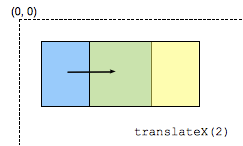`scaleX()` 这个CSS函数是用一个常数因子来修改每个单元点的横坐标，在比例因子是1的情况下该函数是恒等变换。 缩放不是各向同性的，元素的角度也不守恒。

`scaleX(sx)``scale(sx, 1)``scale3d(sx, 1, 1)` 的简写形式。

`scaleX(-1)` 表示通过原点的垂直轴定义轴对称（由 属性指定）。

scaleX(s)

### 参数

s

Cartesian coordinates on ℝ2 Homogeneous coordinates on ℝℙ2 Cartesian coordinates on ℝ3 Homogeneous coordinates on ℝℙ3
$\left(\begin{array}{cc}s& 0\\ 0& 1\end{array}\right)$ $\left(\begin{array}{cc}s0& 0\\ 01& 0\\ 0& 0& 1\end{array}\right)$ $\left(\begin{array}{cc}s0& 0\\ 01& 0\\ 0& 0& 1\end{array}\right)$ $\left(\begin{array}{ccc}s0& 0& 0\\ 01& 0& 0\\ 0& 0& 1& 0\\ 0& 0& 0& 1\end{array}\right)$
`[s 0 0 1 0 0]`

## scaleY()`scaleY()` 这个 CSS函数用一个常数因子修改每个元素点的纵坐标，在比例因子是1的情况下该函数是恒等变换。 缩放不是各向同性的，元素的角度不守恒。

`scaleY(sy)``scale(1, sy)``scale3d(1, sy, 1)` 的简写形式。

`scaleY(-1)` 定义了通过原点的水平轴的轴对称（由 属性指定）。

### 语法

`scaleY(s)`

### 参数

s

Cartesian coordinates on ℝ2 Homogeneous coordinates on ℝℙ2 Cartesian coordinates on ℝ3 Homogeneous coordinates on ℝℙ3
$\left(\begin{array}{c}10\\ 0& s\end{array}\right)$ $\left(\begin{array}{cc}10& 0\\ 0s& 0\\ 0& 0& 1\end{array}\right)$ $\left(\begin{array}{cc}10& 0\\ 0s& 0\\ 0& 0& 1\end{array}\right)$ $\left(\begin{array}{ccc}10& 0& 0\\ 0s& 0& 0\\ 0& 0& 1& 0\\ 0& 0& 0& 1\end{array}\right)$
`[1 0 0 s 0 0]`

## scaleZ()

`scaleZ()` 这个CSS函数是用一个常数因子来修改每个单元点的z轴坐标，在比例因子是1的情况下该函数是恒等变换。 缩放不是各向同性的，元素的角度也不守恒。

`scaleZ(sz)``scale3d(1, 1, sz)` 的简写形式。

`scaleZ(-1)` 定义了通过原点的z轴的轴对称（由 属性指定）。

### 语法

`scaleZ(s)`

### 参数

s

Cartesian coordinates on ℝ2 Homogeneous coordinates on ℝℙ2 Cartesian coordinates on ℝ3 Homogeneous coordinates on ℝℙ3

## skew()

`skew()` 这个CSS函数是一种用于拉伸，或者说是平移，该函数会使得在每个方向上扭曲元素上的每个点以一定角度。这是通过将每个坐标增加一个与指定角度成比例的值和到原点的距离来完成的。离原点越远，拉伸的值就越大。

### 语法

```skew(ax)
skew(ax, ay)
```

### 参数

ax

ay

Cartesian coordinates on ℝ2 Homogeneous coordinates on ℝℙ2 Cartesian coordinates on ℝ3 Homogeneous coordinates on ℝℙ3
$\left(\begin{array}{c}1tan\left(ax\right)\\ tan\left(ay\right)1\end{array}\right)$ $\left(\begin{array}{cc}1tan\left(ax\right)& 0\\ tan\left(ay\right)1& 0\\ 0& 0& 1\\ \end{array}\right)$ $\left(\begin{array}{cc}1tan\left(ax\right)& 0\\ tan\left(ay\right)1& 0\\ 0& 0& 1\end{array}\right)$ $\left(\begin{array}{ccc}1tan\left(ax\right)& 0& 0\\ tan\left(ay\right)1& 0& 0\\ 0& 0& 1& 0\\ 0& 0& 0& 1\end{array}\right)$
`[1 tan(ay) tan(ax) 1 0 0]`

## skewX()

`skewX()` 这个CSS函数是用于水平拉伸，它将元素的每个点在水平方向上扭曲一定角度。这是通过将横坐标增加一个与指定角度成比例的值以及到原点的距离来完成的。离原点越远，拉伸的值就越大。

### 语法

`skewX(a)`

### 参数

a

Cartesian coordinates on ℝ2 Homogeneous coordinates on ℝℙ2 Cartesian coordinates on ℝ3 Homogeneous coordinates on ℝℙ3
$\left(\begin{array}{c}1tan\left(ay\right)\\ 01\end{array}\right)$ $\left(\begin{array}{cc}1tan\left(ay\right)& 0\\ 01& 0\\ 0& 0& 1\end{array}\right)$ $\left(\begin{array}{cc}1tan\left(ay\right)& 0\\ 01& 0\\ 0& 0& 1\end{array}\right)$ $\left(\begin{array}{ccc}1tan\left(ay\right)& 0& 0\\ 01& 0& 0\\ 0& 0& 1& 0\\ 0& 0& 0& 1\end{array}\right)$
`[1 0 tan(a) 1 0 0]`

## skewY()

`skewY()` 这个CSS函数是用于垂直拉伸，它将元素的每个点在垂直方向上扭曲一定角度。这是通过将纵坐标增加一个与指定角度成比例的值以及到原点的距离来完成的。离原点越远，拉伸的值就越大。

### 语法

`skewY(a)`

### 参数

a

Cartesian coordinates on ℝ2 Homogeneous coordinates on ℝℙ2 Cartesian coordinates on ℝ3 Homogeneous coordinates on ℝℙ3
$\left(\begin{array}{c}10\\ tan\left(ax\right)1\end{array}\right)$ $\left(\begin{array}{cc}10& 0\\ tan\left(ax\right)1& 0\\ 0& 0& 1\end{array}\right)$ $\left(\begin{array}{cc}10& 0\\ tan\left(ax\right)1& 0\\ 0& 0& 1\end{array}\right)$ $\left(\begin{array}{ccc}10& 0& 0\\ tan\left(ax\right)1& 0& 0\\ 0& 0& 1& 0\\ 0& 0& 0& 1\end{array}\right)$
`[1 tan(a) 0 1 0 0]`

## translate()`translate()` 这个CSS 函数用于移动元素在平面上的位置。这种变换的特点是矢量的坐标定义了它在每个方向上的移动量。

### 语法

```translate(tx)
translate(tx, ty)
```

### 参数

tx

ty

Cartesian coordinates on ℝ2 Homogeneous coordinates on ℝℙ2 Cartesian coordinates on ℝ3 Homogeneous coordinates on ℝℙ3

$\left(\begin{array}{cc}10& tx\\ 01& ty\\ 0& 0& 1\end{array}\right)$ $\left(\begin{array}{cc}10& tx\\ 01& ty\\ 0& 0& 1\end{array}\right)$ $\left(\begin{array}{ccc}10& 0& tx\\ 01& 0& ty\\ 0& 0& 1& 0\\ 0& 0& 0& 1\end{array}\right)$
`[1 0 0 1 tx ty]`

## translate3d()

`translate3d()` 这个CSS 函数用于移动元素在3D空间中的位置。 这种变换的特点是三维矢量的坐标定义了它在每个方向上的移动量。

### 语法

`translate3d(tx, ty, tz)`

### 参数

tx

ty

tz

Cartesian coordinates on ℝ2 Homogeneous coordinates on ℝℙ2 Cartesian coordinates on ℝ3 Homogeneous coordinates on ℝℙ3

## translateX()`translateX()` 这个CSS 函数用于在平面上水平移动元素。 这个转换的特点是 定义了它的水平移动量。

`translateX(tx)``translate(tx, 0) `的简写形式。

### 语法

`translateX(t)`

### 参数

t

Cartesian coordinates on ℝ2 Homogeneous coordinates on ℝℙ2 Cartesian coordinates on ℝ3 Homogeneous coordinates on ℝℙ3

A translation is not a linear transform in ℝ2 and cannot be represented using a matrix in the cartesian coordinates system.

$\left(\begin{array}{cc}10& t\\ 01& 0\\ 0& 0& 1\end{array}\right)$ $\left(\begin{array}{cc}10& t\\ 01& 0\\ 0& 0& 1\end{array}\right)$ $\left(\begin{array}{ccc}10& 0& t\\ 01& 0& 0\\ 0& 0& 1& 0\\ 0& 0& 0& 1\end{array}\right)$
`[1 0 0 1 t 0]`

## translateY()`translateY()` 这个CSS 函数用于在平面上垂直移动元素。 这个转换的特点是通过 定义了它的垂直移动量。

`translateY(ty)``translate(0, ty)`的简写形式。

### 语法

```translateY(t)
```

### 参数

t

Cartesian coordinates on ℝ2 Homogeneous coordinates on ℝℙ2 Cartesian coordinates on ℝ3 Homogeneous coordinates on ℝℙ3

A translation is not a linear transform in ℝ2 and cannot be represented using a matrix in the cartesian coordinates system.

$\left(\begin{array}{cc}10& 0\\ 01& t\\ 0& 0& 1\end{array}\right)$ $\left(\begin{array}{cc}10& 0\\ 01& t\\ 0& 0& 1\end{array}\right)$ $\left(\begin{array}{ccc}10& 0& 0\\ 01& 0& t\\ 0& 0& 1& 0\\ 0& 0& 0& 1\end{array}\right)$
`[1 0 0 1 0 t]`

## translateZ()

`translateZ()` 这个CSS 函数用于3D空间的z轴方向移动元素， 这个转换的特点是通过 定义了它的z轴方向移动量。

`translateZ(tz)``translate3d(0, 0, tz) `的简写形式。

### 语法

`translateZ(t)`

### 参数

t

Cartesian coordinates on ℝ2 Homogeneous coordinates on ℝℙ2 Cartesian coordinates on ℝ3 Homogeneous coordinates on ℝℙ3